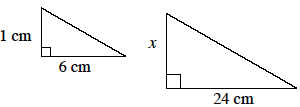### Home > CC2MN > Chapter 4 > Lesson 4.4.1 > Problem4-129

4-129.The two triangles at right are similar.

1. Find $x$.

What would the scale factor be between these two shapes?
Since $1$ cm is the corresponding side, multiply it by the scale factor to get $x$.

$x=4$ cm

2. Find the area of the smaller triangle.

Use the formula for the area of a triangle: $\frac{1}{2}\left(6\ \text{cm}\right)\left(1\ \text{cm}\right)$

3. Based on ratios of similarity, find the area of the large triangle.

When using ratios of similarity to scale a shape, the area of the old shape is multiplied by the scale factor squared to get the area of the new shape.

$3(4)^2=48$ cm2

4. Find the area of the larger triangle by using the formula for the area of a triangle.

Use the formula for the area of a triangle: $\frac{1}{2}\left(\text{base}\right)\left(\text{height}\right)$

5. Verify that your answers to (c) and (d) are the same.

Did you get the same answer? Make sure to show your work.